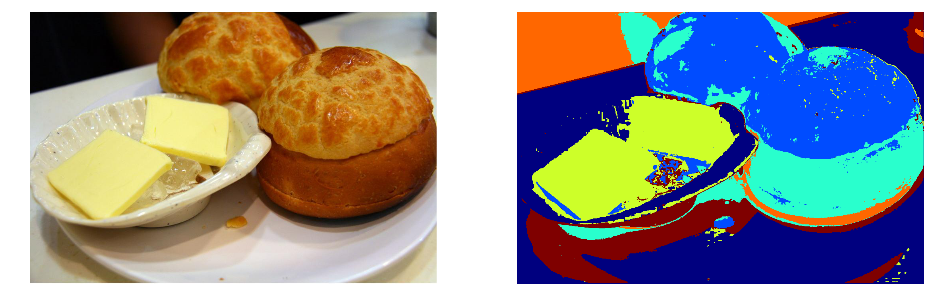×

Search anything:

# Mean Shift Clustering Algorithm

#### Algorithms clustering algorithm mean shift clustering Machine Learning (ML) data miningGet this book -> Problems on Array: For Interviews and Competitive Programming

Mean Shift clustering algorithm is an unsupervised clustering algorithm that groups data directly without being trained on labelled data. The nature of the Mean Shift clustering algorithm is heirarchical in nature, which means it builds on a heirarchy of clusters, step by step.

## Understanding the algorithm

Let's understand the mean shift algorithm with the help of set of data points in a 2D plane. As it is a clustering algorithm, our final goal is to represent this unordered data in an organized way, and divide it into clusters.

Mean Shift essentially starts off with a kernel, which is basically a circular sliding window. The bandwidth, i.e. the radius of this sliding window will be pre-decided by the user.

A very high level view of the algorithm can be:
STEP 1: Pick any random point, and place the window on that data point.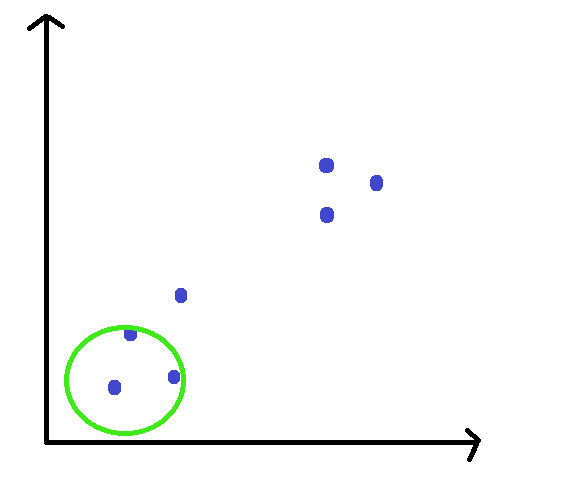STEP 2: Calculate the mean of all the points lying inside this window.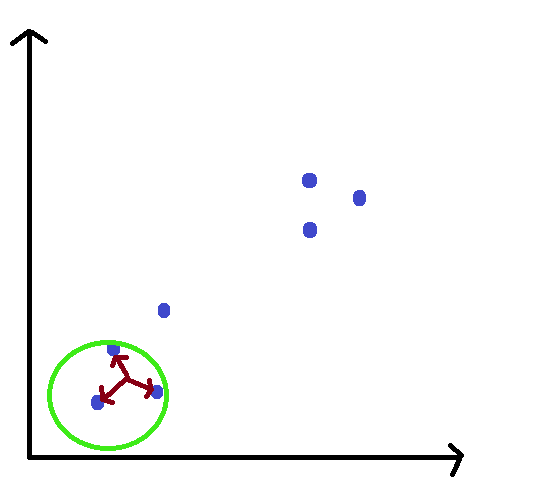STEP 3: Shift the window, such that it is lying on the location of the mean.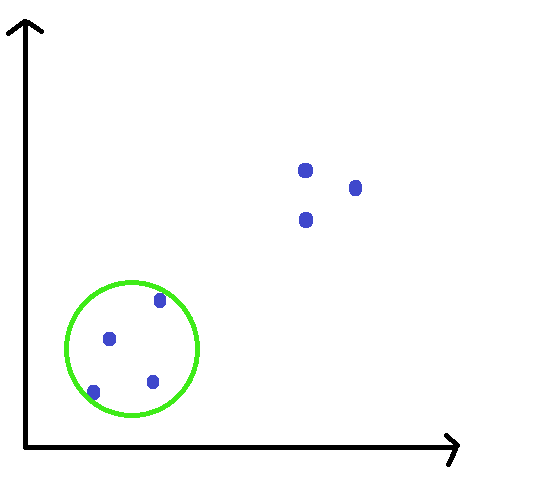STEP 4: Repeat till convergence.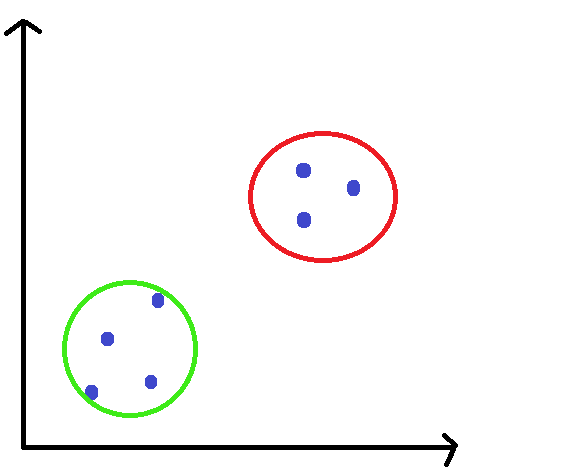What we're trying to achieve here is, to keep shifting the window to a region of higher density. This is why, we keep shifting the window towards the centroid of all the points in the window. This feature of Mean Shift algorithm describes it's property as a hill climb algorithm.

Mean shift clustering aims to discover “blobs” in a smooth density of samples. It is a centroid-based algorithm, which works by updating candidates for centroids to be the mean of the points within a given region. These candidates are then filtered in a post-processing stage to eliminate near-duplicates to form the final set of centroids. - Official sklean documentation

## Intuition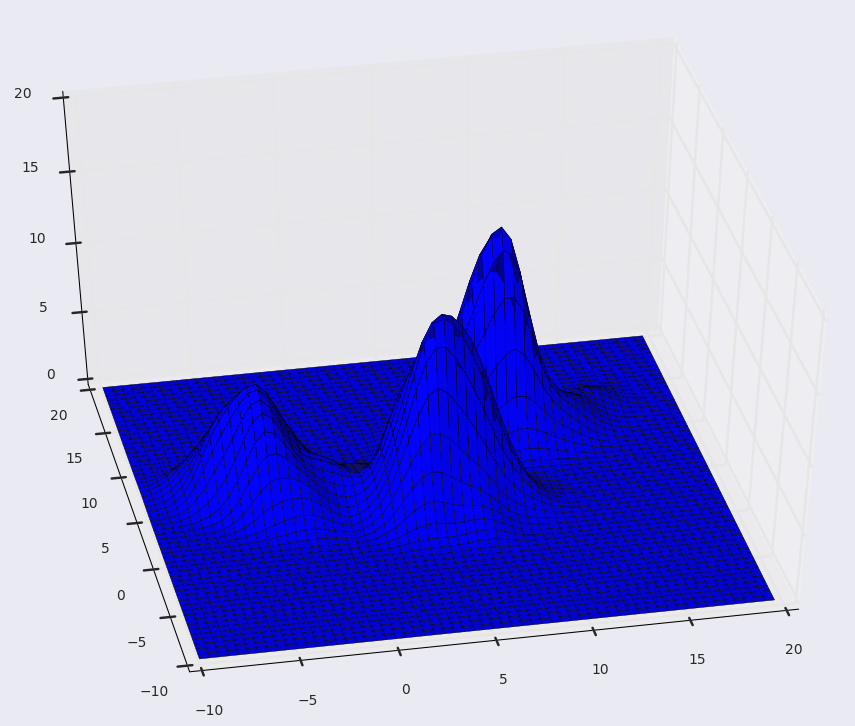We can understand this algorithm by thinking of our data points to be represented as a probability density function. Naturally, in a probability function, higher density regions will correspond to the regions with more points, and lower density regions will correspond to the regions with less points.

In clustering, we need to find clusters of points, i.e the regions with a lot of points together. More points together mean higher density. Hence, we observe that clusters of points are more like the higher density regions in our probability density function.

So, we must iteratively go from lower density to higher density regions, in order to find our clusters.

This is exactly what we aim to achieve with the Mean Shift algorithm, iteratively finding the high density regions, and shifting our window in that direction, until we reach convergence.

## What exactly does convergence mean?

In the earlier part of this article, we discussed the procedure of Mean Shift, and implied that we stop at convergence. Here, we will discuss what our stopping condition will be for the algorithm.

Mean Shift algorithm proceeds the STEPS 1-4, and finds a 'final' window location for each of the data points. For each iteration, we find the centroid of all the points in the window, shift the window accordingly, and repeat. However, after a sufficient number of steps, the position of the centroid of all the points, and the current location of the window will coincide. This is when we reach convergence, as no new points are added to our window in this step.

Also, one thing we can straightaway notice, is that not all points will have a different location of the 'final' window. In fact, all points lying in the same cluster, will end up with more or less the same final steps, given a reasonable bandwidth value. Hence, when multiple windows overlap, we preserve only the window containing the most points. Clusters are then formed according to how the data points lie in the windows.

## Pros

• Mean Shift is quite better at clustering as compared to K Means, mainly due to the fact that we don't need to specify the value of 'K', i.e. the number of clusters.
• Output of mean shift is not dependent on initialization
• The algorithm only takes one input, the bandwidth of the window.

## Cons

• Mean Shift performs a lot of steps, so it can be computationally expensive, with a time complexity of O(n(squared))
• The selection of the bandwidth itself can be non-trivial.
• If the bandwidth is too small, enough data points may be missed, and convergence might never be reached.
• If the bandwidth is too large, a few clusters may be missed completely.

## Complexity

The Mean Shift clustering algorithm can be computationally expensive for large datasets, because we have to iteratively follow our procedure for each data point.

It has a time complexity of O(n(squared)), where n is the number of data points.

## Applications

Mean Shift clustering algorithm is mainly applied in Computer Vision problems.

Popular applications include:
Image Processing
Video Tracking
Image S
egmentation.

Mean Shift algorithm would also have been really effective in big data problems, if it were not so computationally expensive. Currently, there is ongoing research aiming to find convergence faster, which would solve this problem.

Here is an example of Mean-Shift clustering for a Computer Vision problem.The image is divided into clusters, based on the colour of objects in the image.